# Irodov Solutions: Relativistic Mechanics - 1 Notes | Study I. E. Irodov Solutions for Physics Class 11 & Class 12 - JEE

## JEE: Irodov Solutions: Relativistic Mechanics - 1 Notes | Study I. E. Irodov Solutions for Physics Class 11 & Class 12 - JEE

The document Irodov Solutions: Relativistic Mechanics - 1 Notes | Study I. E. Irodov Solutions for Physics Class 11 & Class 12 - JEE is a part of the JEE Course I. E. Irodov Solutions for Physics Class 11 & Class 12.
All you need of JEE at this link: JEE

Q.340. A rod moves lengthwise with a constant velocity v relative to the inertial reference frame K. At what value of v will the length of the rod in this frame be η = 0.5% less than its proper length?

Solution. 340. From the formula for length contraction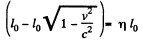So,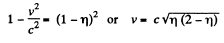Q.341. In a triangle the proper length of each side equals α. Find the perimeter of this triangle in the reference frame moving relative to it with a constant velocity V along one of its
(a) bisectors;
(b) sides.

Solution. 341. (a) In the frame in which the triangle is at rest the space coordinates of the vertices are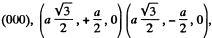all measured at the same time t. In the moving frame the corresponding coordinates at time t' are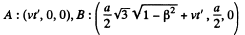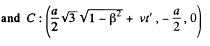The perimeter P is then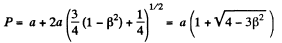(b) The coordinates in the first frame are shown at time t. The coordinates in the moving frame are,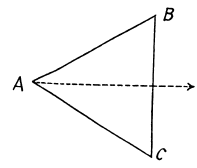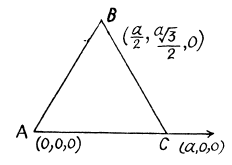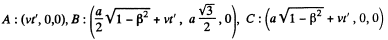The perimeter P is then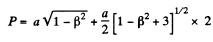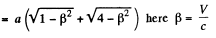Q.342. Find the proper length of a rod if in the laboratory frame of reference its velocity is v = c/2, the length l = 1.00 m, and the angle between the rod and its direction of motion is θ = 45°.

Solution. 342. In the rest frame, the coordinates of the ends of the rod in terms of proper length l0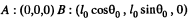at time t. In the laboratory frame the coordinates at time t' are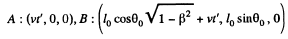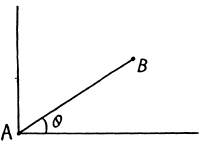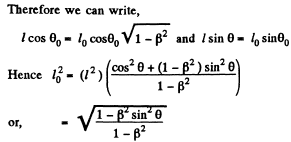Q.343.  A stationary upright cone has a taper angle θ =. 45°, and the area of the lateral surface So  = 4.0 m2. Find: (a) its taper angle; (b) its lateral surface area, in the reference frame moving with a velocity v = (4/5)c along the axis of the cone.

Solution. 343. In the frame K in which the cone is at rest the coordinates of A are (0,0,0) and of B are (h, h tan θ, 0) . In the frame K , which is moving with velocity v along the axis o f the cone, the coordinates of A and B at time t' are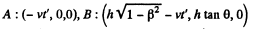Thus the taper angle in the frame K is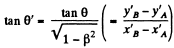and the lateral surface area is,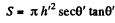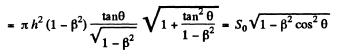Here S0 - πh2 secθ tanθ is the lateral surface area in the rest frame and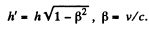Q.344. With what velocity (relative to the reference frame K) did the clock move, if during the time interval t = 5.0 s, measured by the clock of the frame K, it became slow by Δt = 0.10 s?

Solution. 344. Because of time dilation, a moving clock reads less time. We write,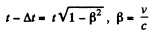Thus,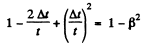or,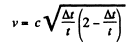Q.345. A rod flies with constant velocity past a mark which is stationary in the reference frame K. In the frame K it takes Δt = 20 ns for the rod to fly past the mark. In the reference frame fixed to the rod the mark moves past the rod for Δt' = 25 ns. Find the proper length of the rod.

Solution. 345. In the frame K the length l of the rod is related to the time of flight Δt by

l = v Δt

In the reference frame fixed to the rod (frame K')the proper length l0 of the rod is given by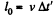But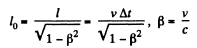Thus,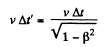So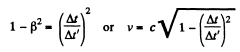and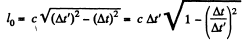Q.346.  The proper lifetime of an unstable particle is equal to Δt0  = 10 ns. Find the distance this particle will traverse till its decay in the laboratory fraine of reference, where its lifetime is equal to Δt = 20 ns.

Solution. 346. The distance travelled in the laboratory frame of reference is vΔ t where v is the velocity of the particle. But by time dilation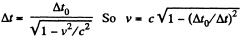Thus the distance traversed is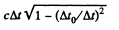Q.347. In the reference frame K a muon moving with a velocity v = 0.990c travelled a distance l = 3.0 km from its birthplace to the point where it decayed. Find:
(a) the proper lifetime of this muon;
(b) the distance travelled by the muon in the frame K "from the muon's standpoint".

Solution. 347. (a) If τ0 is the proper life time of the muon the life time in the moving frame is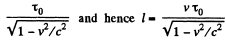Thus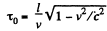(The words "from the muon’s stand point" are not part of any standard terminology)

Q.348. Two particles moving in a laboratory frame of reference along the same straight line with the same velocity v = (3/4)c strike against a stationary target with the time interval Δt = 50 ns. Find the proper distance between the particles prior to their hitting the target.

Solution. 348. In the frame K in which the particles are at rest, their positions are A and B whose coordinates may be taken as,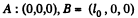In the frame K' with respect to which K is moving with a velocity v the coordinates of A and B at time t' in the moving frame are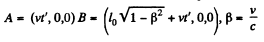Suppose B hits a stationary target in K' after time t'B while A hits it after time tB + Δt. Then,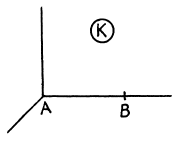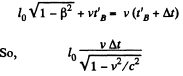Q.349. A rod moves along a ruler with a constant velocity. When the positions of both ends of the rod are marked simultaneously in the reference frame fixed to the ruler, the difference of readings on the ruler is equal to Δx1  = 4.0 m. But when the positions of the rod's ends are marked simultaneously in the reference frame fixed to the rod, the difference of readings on the same ruler is equal to Δx2 = 9.0 m. Find the proper length of the rod and its velocity relative to the ruler.

Solution. 349. In the reference frame fixed to the ruler the rod is moving with a velocity v and suffers Lorentz contraction. If l0 is the proper length of the rod, its measured length will be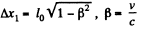In the reference frame fixed to the rod the ruler suffers Lorentz contraction and we must have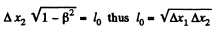and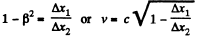Q.350. Two rods of the same proper length l0  move toward each other parallel to a common horizontal axis. In the reference frame fixed to one of the rods the time interval between the moments, when the right and left ends of the rods coincide, is equal to Δt. What is the velocity of one rod relative to the other?

Solution. 350. The coordinates of the ends of the rods in the frame fixed to the left rod are shown. The points B and D coincides when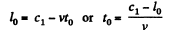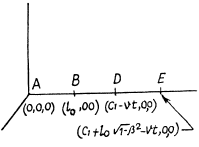The points A and E coincide when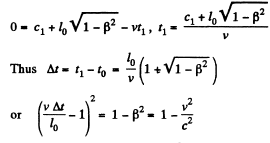From this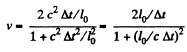Q.351. Two unstable particles move in the reference frame K along a straight line in the same direction with a velocity v = 0.990c. The distance between them in this reference frame is equal to l = 120 m. At a certain moment both particles decay simultaneously in the reference frame fixed to them. What time interval between the moments of decay of the two particles will be observed in the frame K? Which particle decays later in the frame K?

Solution. 351. In K0, the rest frame of the particles, the events corresponding to the decay of the particles are,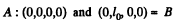In the reference frame Kf the corresponding coordintes are by Lorentz transformation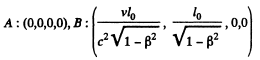Now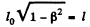by Lorentz Fitzgerald contraction formula. Thus the time lag of the decay time of B is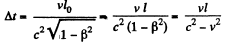B decays later (B is the forward particle in the direction of motion)

Q.352. A rod AB oriented along the x axis of the reference frame K moves in the positive direction of the x axis with a constant velocity v. The point A is the forward end of the rod, and the point B its rear end. Find:
(a) the proper length of the rod, if at the moment tA the coordinate of the point A is equal to xA, and at the moment tB the coordinate of the point B is equal to xB;
(b) what time interval should separate the markings of coordinates of the rod's ends in the frame K for the difference of coordinates to become equal to the proper length of the rod.

Solution. 352. (a) In the reference frame K with respect to which the rod is moving with velocity v, the coordinates of A and B are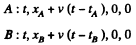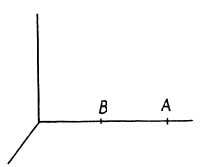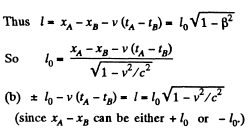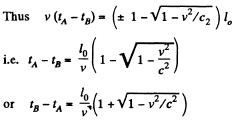Q.353. The rod A'B' moves with a constant velocity v relative to the rod AB (Fig. 1.91). Both rods have the same proper length l0 and at the ends of each of them clocks are mounted, which are synchronized pairwise: A with B and A' with B'. Suppose the moment when the clock B' gets opposite the clock A is taken for the beginning of the time count in the reference frames fixed to each of the rods. Determine:

(a) the readings of the clocks B and B' at the moment when they are opposite each other;
(b) the same for the clocks A and A'.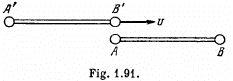Solution. 353.  At the instant the picture is taken the coordintes of A, B, A', B' in the rest frame of AB are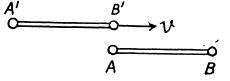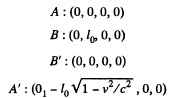In this frame the coordinates o f B ' at other times are B': (t, vt, 0, 0). So B ' is opposite to B at time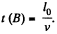In the frame in which B', A' is at rest the time corresponding this is by Lorentz tranformation.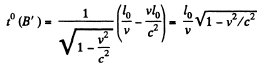Similarly in the rest frame of A, B, te coordinates of A at other times are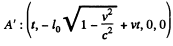A' is opposite to A at time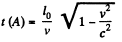The corresponding time in the frame in which A', B' are at rest is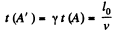Q.354. There are two groups of mutually synchronized clocks K and K' moving relative to each other with a velocity v as shown in Fig. 1.92. The moment when the clock A' gets opposite the clock A is taken for the beginning of the time count. Draw the approximate position of hands of all the clocks at this moment "in terms of the K clocks"; "in terms of the K' clocks".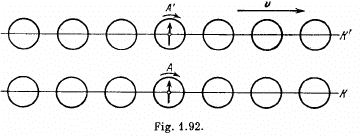Solution. 354. By Lorentz transformation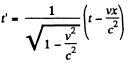So at time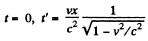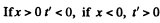and we get the diagram given below "in terms o f th eif-clock ".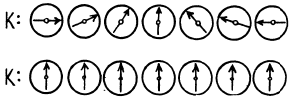The situation in terms of the K' clock is reversed.

Q.355. The reference frame K' moves in the positive direction of the x axis of the frame K with a relative velocity V. Suppose that at the moment when the origins of coordinates O and O' coincide, the clock readings at these points are equal to zero in both frames. Find the displacement velocity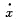of the point (in the frame K) at which the readings of the clocks of both reference frames will be permanent- ly identical. Demonstrate that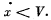Solution. 355. Suppose x (t) is the locus of points in the frame K at which the readings of the clocks of both reference system are permanently identical then by Lorentz transformation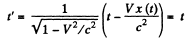So differentiatin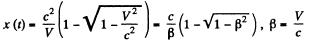Let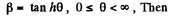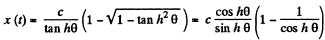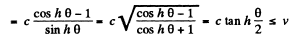(tan h θ is a monotonically increasing function of θ)

The document Irodov Solutions: Relativistic Mechanics - 1 Notes | Study I. E. Irodov Solutions for Physics Class 11 & Class 12 - JEE is a part of the JEE Course I. E. Irodov Solutions for Physics Class 11 & Class 12.
All you need of JEE at this link: JEEUse Code STAYHOME200 and get INR 200 additional OFF

## I. E. Irodov Solutions for Physics Class 11 & Class 12

111 docs

Track your progress, build streaks, highlight & save important lessons and more!

,

,

,

,

,

,

,

,

,

,

,

,

,

,

,

,

,

,

,

,

,

;